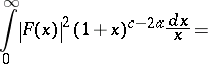# Olevskii transform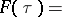(a1)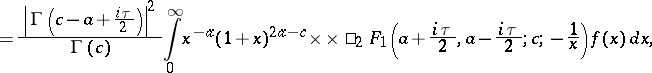whereis a Gauss hypergeometric function. It was introduced by M.N. Olevskii in [a1].

Letting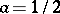, one obtains the Mehler–Fock transform. By changing the variable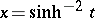and the respective parameters of the Gauss function, one obtains the Fourier–Jacobi transform [a2].

One can show that the Olevskii transform is the composition of the Kontorovich–Lebedev transform and the Hankel transform (cf. Integral transform; Hardy transform).

The Gauss function in the integral (a1) is the hypergeometric series for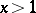and for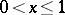one can understand it as an analytic continuation, which can be obtained from the Mellin–Barnes integral representation [a3].

The following integral transform is also called the Olevskii transform. It is an integral over the indexof the Gauss function,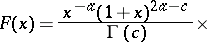(a2)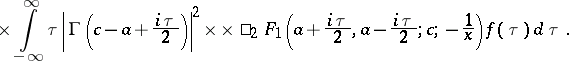Here,is an arbitrary odd function belonging to the space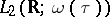, whereThe transform (a2) maps this space onto the spaceand the Parseval equality holds: